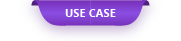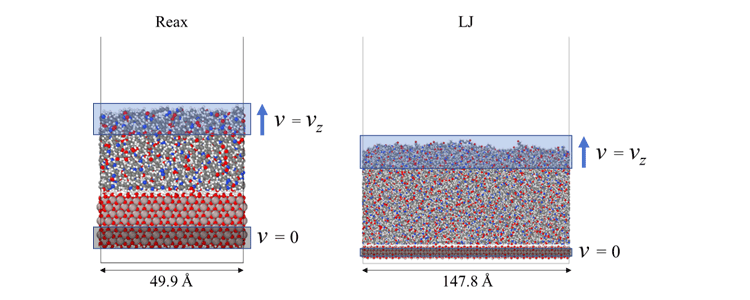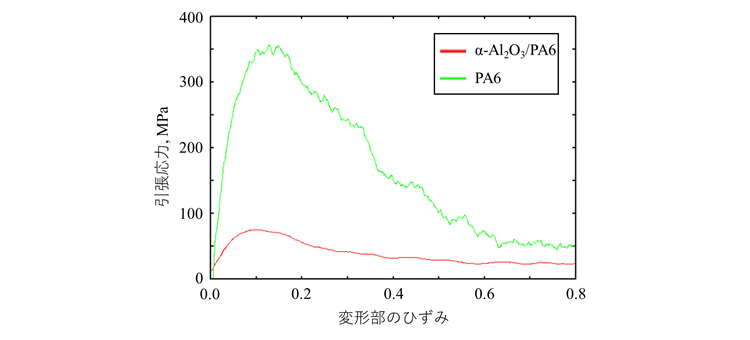In structures such as automobile bodies, multi-materialization that uses different materials in the right place is attracting attention.

# Metal / resin interface energy and potential study in tensile simulation

#### Overview

In structures such as automobile bodies, multi-materialization that uses different materials in the right place is attracting attention. In multi-materialization, the joints of different materials are the weak points, so advances in dissimilar material joining technology are required. Especially in metal-resin bonding, nanoscale interactions at the bonding interface have a significant effect on bond strength. Therefore, since it is not possible to elucidate the phenomenon of the bond in detail in the experiment, research using molecular simulation technology is underway.
First of all, in order to verify which potential should be used, molecular dynamics is used for the peeling of the joint between Al, which is a light metal, and PA6, which is used as a base material for thermoplastic CFRP, using the Reax potential and LJ potential. ( MD ) Performed using simulation.

#### Method of calculation

In the bonding of Al and PA 6, it was assumed that the bonding was performed at the interface between the Al oxide film (α-Al 2 O 3 ) and PA 6, and the peeling simulation of the smooth interface between α-Al 2 O 3 and PA 6 was performed. The potential used was Reax potential and LJ potential. The α-Al 2 O 3 / PA 6 interface was created by melting PA 6 on the α-Al 2 O 3 surface by heating simulation. The peeling calculation was performed by fixing the atoms in a part (gray part) of α-Al 2 O 3 and giving the atoms in the upper part (blue part) of PA6 an upward constant velocity v z . LAMMPS was used for these calculations.

#### Calculation condition

##### model

PA6 : Number of polymerizations n = 10, Specific gravity: 1.13
α-Al 2 O 3 : Termination of surface oxygen with hydrogen

#### Calculation result

Figure 2 shows snapshots of simulations using the Reax potential and LJ potential (strain amount of deformed part: 0.0, 0.1, 0.2, 1.0), respectively. Regardless of which potential is used, it can be seen that fracture occurs due to peeling and cracking of the resin at the interface.

The SS curves calculated for each potential are shown in Fig. 3 and Fig. 4. The calculation by Reax potential did not give the SS curve as shown by general resin. It is thought that this is because the model size is small due to the heavy calculation in the Reax potential, and the resin is too long for the bulk size. On the other hand, the calculation based on the LJ potential yielded an SS curve with a strain of about 0.1.

As shown in Figure 5, the peel strength depends on the peeling speed and increases as the peeling speed increases. It is thought that this is because the faster the peeling speed, the more the tension progresses without relaxing the system, and the system follows an energetically unstable trajectory. In this calculation, the pulling speed is much faster than the actual system, so it is considered that the tensile stress is calculated larger than the actual one.

In addition, in order to clarify the effect of the α-Al 2 O 3 / PA6 interface , Fig. 6 shows the SS curve obtained from the peeling simulation of the strain rate of 1.7 × 10 10 and the tensile simulation of PA6 with the same strain rate. Shown. As mentioned above, since the calculation was performed at a faster tensile speed than in the experiment, the strength of the resin showed a value larger than the experimental value (60 to 100MP), but it can be seen that the breaking strength decreased due to the presence of the interface.Fig. 6 SS curve of α-Al 2 O 3 / PA6 interface model and PA6 bulk model (strain rate 1.7 × 10 10 / s)

The bonding work was calculated from the energy difference between the bonded state and the separated state of α-Al 2 O 3 and PA 6, and is shown in Table 2.

The calculated glue work showed a slightly higher value than it really is. This may be due to the small size of the model, which does not include large defects such as those present at the actual interface, and the accuracy of the potential used.

Next, we compared it with the DFT calculation to confirm the validity of each potential. The comparison with the DFT calculation was made by comparing the change in relative energy when the distance between α-Al 2 O 3 and the PA6 monomer was changed as shown in Fig. 7 .